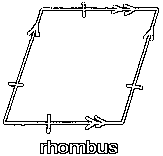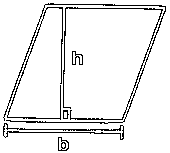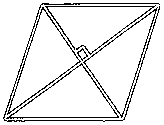# The RhombusThe rhombus is a quadrilateral with all sides equal in length. Notice that it is a parallelogram, since opposite sides are parallel.Since the rhombus is a parallelogram, you find its area using the same method that you would use for a parallelogram.

The length of the base of the rhombus is the length of one of its sides, here shown with 'b'.

The height is the perpendicular distance between opposite sides, here shown with an 'h'.

The area of the rhombus is equal to its base times its height, or:

area = bhThe diagonals of a rhombus are perpendicular.A square is a rhombus since it is a quadrilateral with all sides equal.

Custom Search